RS Aggarwal Class 7 Solutions Chapter 13 - Lines And Angles

RS Aggarwal Class 7 Chapter 13 - Lines And Angles Solutions Free PDF

A line without any beginning or ending points, which can extend indefinitely. It is one of the basic representations of straight objects without negligible width and depth. The different types of lines are:

1. Straight lines
2. Perpendicular lines
3. Parallel lines

When two lines emerge from a common point, pointing towards a certain direction is titled as an angle. It is represented as ‘∠ ‘. The instrument used for measuring an angle is called a protractor.

The different types of angles are:

1. Straight angle
2. Obtuse angle
3. Acute angle
4. Right angle
5. Complete angle
6. Linear pairs of angles
7. Vertical angle
8. Supplementary angle
9. Complementary angle
10. Corresponding angles
11. Alternate interior angles
12. Alternate exterior angles
14. Vertically opposite angles

The RS Aggarwal Class 7 Solutions Chapter 13 Lines and Angles can be found below:

Q1: Find the complement of each of the following angles:

(i) $35^{.}$

(ii) $47^{.}$

(iii) $60^{.}$

(iv) $73^{.}$

Sol:

(i) The given angle measures $35^{.}$.

Let the measure of its complement be $x^{.}$.

x + $35^{.}$ = $90^{.}$

or, x = $90^{.} – 35^{.} = 55^{.}$

Hence, the complement of the given angle will be $55^{.}$

(ii) The given angle measures $47^{.}$.

Let the measure of its complement be $x^{.}$.

x + $47^{.}$ = $90^{.}$

or, x = $90^{.} – 47^{.} = 43^{.}$

Hence, the complement of the given angle will be $43^{.}$

(iii) The given angle measures $47^{.}$.

Let the measure of its complement be $x^{.}$.

x + $60^{.}$ = $90^{.}$

or, x = $90^{.} – 60^{.} = 30^{.}$

Hence, the complement of the given angle will be $30^{.}$

(iv) The given angle measures $73^{.}$.

Let the measure of its complement be $x^{.}$.

x + $73^{.}$ = $90^{.}$

or, x = $90^{.} – 73^{.} = 17^{.}$

Hence, the complement of the given angle will be $17^{.}$

Q2: Find the supplement of each of the following angles:

(i) $80 ^{.}$

(ii) $54 ^{.}$

(iii) $105 ^{.}$

(iv) $123 ^{.}$

Sol:

(i) The given angle measures $80 ^{.}$.

Let the measure of its complement be $x^{.}$.

x + $80^{.}$ = $180^{.}$

or, x = $180^{.} – 80^{.} = 100^{.}$

Hence, the supplement of the given angle will be $100^{.}$

(ii) The given angle measures $54 ^{.}$.

Let the measure of its complement be $x^{.}$.

x + $54^{.}$ = $180^{.}$

or, x = $180^{.} – 54^{.} = 126^{.}$

Hence, the supplement of the given angle will be $126^{.}$

(iii) The given angle measures $105 ^{.}$.

Let the measure of its complement be $x^{.}$.

x + $105^{.}$ = $180^{.}$

or, x = $180^{.} – 105^{.} = 75^{.}$

Hence, the supplement of the given angle will be $75^{.}$

(iv) The given angle measures $123 ^{.}$.

Let the measure of its complement be $x^{.}$.

x + $123^{.}$ = $180^{.}$

or, x = $180^{.} – 123^{.} = 57^{.}$

Hence, the supplement of the given angle will be $57^{.}$

Q3: Among two supplementary angles, the measure of the larger angle is $36 ^{.}$ more than the measure of the smaller. Find their measures.

Sol:

Let the two supplementary angles be $x^{.}$. and $(180 – x)^{.}$.

Since it is given that the measure of the larger angle is $36 ^{.}$ more than the smaller angle, let the larger angle be $x^{.}$.

Therefore, $(180 – x)^{.} + 36^{.} = x^{.}$

or 216 = 2x

Or x = 108

Thus larger angle = $108^{.}$

Smaller angle = $(108 – 36)^{.}$

= $72^{.}$

Q4:Find the angle which is equal to its supplement.

Sol:

Let the measure of the required angle be x.

Since it is its own supplement.

x + x = $180^{.}$

or 2x = $180^{.}$

or x = $90^{.}$

Therefore the required angle is $90^{.}$.

Q5: Can two angles be supplementary if both of them are:

(i) acute?                          (ii) Obtuse?                          (iii) right?

Sol:

(i) No. If both the angles are acute i.e. less than $90^{.}$, they cannot be supplement as their supplement will always be less than $180 ^{.}$.

(ii)  No. If both the angles are obtuse i.e. more than $90^{.}$, they cannot be supplement as their sum will always be more than $180 ^{.}$.

(iii) Yes. If both the angles are right i.e. they both measure $90^{.}$, then they form a supplementary pair.

$90^{.} + 90^{.} = 180^{.}$

Q6: In the given figure, AOB is a straight line and the ray OC stands on it. If $\angle AOC = 64^{.}$ and $\angle BOC = x^{.}$, find the value of x.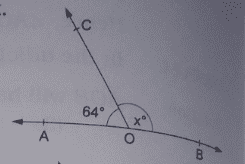Sol:

By linear pair property,

$\angle AOC + angle COB = 180^{.}$

$64^{.} + angle COB = 180^{.}$

$\angle COB = 180^{.} – 64^{.} = 116^{.}$

Therefore x = $116^{.}$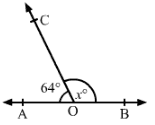Q7: In the given figure, AOB is a straight line and the ray OC stands on it. If $\angle AOC = (2 x – 10)^{.}$ and $\angle BOC = (3 x + 20)^{.}$, find the value of x. Also, find $\angle AOC ;; and ;; angle BOC$.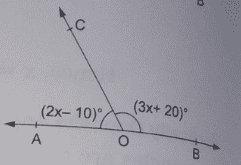Sol:

By linear property:

$\angle AOC + angle BOC = 180^{.}$

$(2 x – 10)^{.} + (3x + 20) ^{.} = 180^{.}$            (Given)

Or 5x + 10 = 180

Or x = $34^{.}$

Therefore $angle AOC = (2 x – 10) ^{.} = (2 times 34 – 10) ^{.} = 58^{.}$

$angle BOC = (3 x + 20) ^{.} = (3 times 34 + 20) ^{.} = 122^{.}$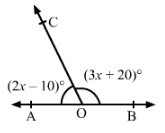Q8: In the given figure, AOB is a straight line and the ray OC and OD stand on it. If $\angle AOC = 65^{.}$ , $\angle BOC = 70^{.}$ and $\angle COD = x^{.}$, find the value of x.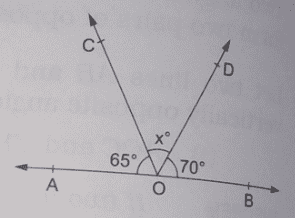Sol:

Since AOB is a straight line, we have:

$\angle AOC + angle BOD + angle COD = 180^{.}$

or, $65^{.} + 70 ^{.} + x ^{.} = 180^{.}$         (Given)

Or, $135 ^{.} + x ^{.} = 180^{.}$

Or, $x ^{.} = 45^{.}$

Thus, the value of x is $45^{.}$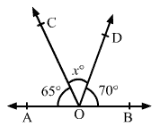Q9: In the given figure, two straight lines AB and CD intersect at a point O.

If $\angle AOC = 42^{.}$, find the measure of each of the angles:

(i)  $\angle AOD$

(ii) $\angle BOD$

(iii) $\angle COB$

Sol: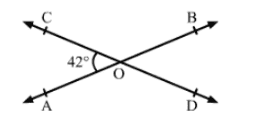Sol:

AB and CD intersect at O and CD is a straight line.

(i) $\angle COA + angle AOD = 180^{.}$       (Linear pair)

$42^{.} + angle AOD = 180^{.}$

$angle AOD = 138^{.}$

(ii) $\angle COA ;; and ;; angle BOD$ are vertically opposite angles.

Therefore, $angle COA = angle BOD = 42^{.}$    ( from (i) )

(iii) $\angle COB ;; and ;; angle AOD$ are vertically opposite angles.

Therefore, $\angle COB = angle AOD = 138^{.}$    ( from (i) )

Q10: In the given figure, two straight lines PQ and RS intersect at O. If $angle POS = 114^{.}$, find the measure of each of the angles:

(i)  $\angle POR$

(ii) $\angle ROQ$

(iii) $\angle QOS$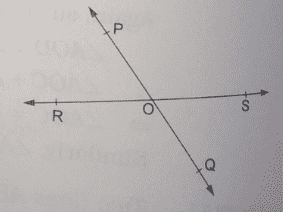Sol:

(i) $angle POS + angle PQR = 180^{.}$      (Linear pair)

Or $114^{.} + angle PQR = 180^{.}$

Or $\angle PQR = 180^{.} – 114^{.} = 66^{.}$

(ii) Since $angle POS ;; and ;; angle QOR$ are vertically opposite angles, they are equal.

Therefore, $\angle QOR = 114^{.}$

(iii) Since $angle POR ;; and ;; angle QOS$ are vertically opposite angles, they are equal.

Therefore, $\angle QOS = 66^{.}$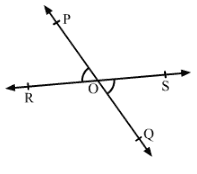Q11: In the given figure, ray OA, OB, OC and OD are such that $\angle AOB = 56^{.}$ , $\angle BOC = 100^{.}$, $\angle COD = x^{.}$ and $\angle DOA = 74^{.}$, find the value of x.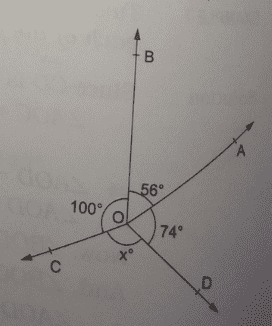Sol: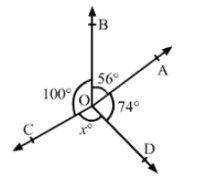Sum of all the angles around a point is $360^{.}$

Therefore, $\angle AOB + angle BOC + angle COD + angle DOA = 360^{.}$

or, $56^{.} + 100^{.} + x^{.} + 74^{.} = 360^{.}$      (Given)

or, $230^{.} + x^{.} = 360^{.}$

or, $x^{.} = 360^{.} – 230^{.}$

or, x = $130^{.}$

Practise This Question

Find the value of X in the given figure: